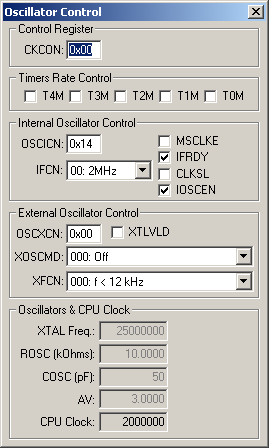Products Download Events Support Videos All Product Families ARM7, ARM9, and Cortex-M3 Products C16x, XC16x, and ST10 Products C251 and 80C251 Products Cx51 and 8051 Products Modified Anytime In the Last 7 Days In the Last 14 Days In the Last 30 Days In the Last 90 Days In the Last 6 Months

# Peripheral Simulation

For Silicon Laboratories, Inc. C8051F001 — Clock and Timer Rate Control

Simulation support for this peripheral or feature is comprised of:

• Dialog boxes which display and allow you to change peripheral configuration.
• VTREGs (Virtual Target Registers) which support I/O with the peripheral.

These simulation capabilities are described below.

### Oscillator Control DialogThe Oscillator Control Dialog controls the oscillator and timer rate control for this controller. With this dialog, you control the internal and external clocking to change clock and timer speeds.

Control Register

• CKCON contains the Timer Rate Control bits.

Timer Rate Control

• TnM (Timer n Clock Select) uses the system clock for Timer n, when set. When reset, Timer n uses the system clock divided by 12.

Internal Oscillator Control

• OSCICN (Internal Oscillator Control Register) controls the internal oscillator and contains the following bits:
• MSCLKE (Missing Clock Enable Bit) is set to enable the Missing Clock Detector. If a clock is missing for more than 100 µsec, a reset is triggered.
• IFRDY (Internal Oscillator Frequency Ready Flag) is set when the internal oscillator frequency is running at the speed specified by the IFCN bits.
• CLKSL (System Clock Source Select Bit) is set to use the external clock as the system clock. If reset, the internal clock is used.
• IOSCEN (Internal Oscillator Enable Bit) is set to enable the internal oscillator.
• IFCN (Internal Oscillator Frequency Control Bits) selects the internal oscillator frequency (2, 4, 8 or 16 MHz).

Internal Oscillator Control

• OSCXCN (External Oscillator Control Register) controls the external oscillator and contains the following bits:
• XTLVLD (Crystal Oscillator Valid Flag) is set when the crystal oscillator is running and stable.
• XOSCMD (External Oscillator Mode Bits) control the system clock divisor and the oscillator mode (RC/C).
• XFCN (External Oscillator Frequency Control Bits) selects the external oscillator frequency.

Oscillators and CPU Clock

• XTAL Freq. contains the crystal frequency.
• ROSC(kOhms) in RC circuit mode, contains the pull-up resistor value in kOhms.
• COSC(pF) in RC or C mode, contains the capacitance value in picofarads.
• AV is the analog power supply value in volts.
• CPU Clock displays the calculated clock rate.

### CLOCK VTREGData Type: unsigned long

The CLOCK VTREG contains the speed of the microcontroller instruction clock (in Hertz). This is effectively the number of 1-cycle instructions that the simulated microcontroller can execute each second. This number is derived by dividing the oscillator frequency (specified by the XTAL VTREG) by a fixed number (12 on standard 8051 devices). The CLOCK VTREG can only be read. It cannot be written. It is changed by changing the value of the XTAL VTREG or by changing the clock divisor (which is not available on all devices). Typing the CLOCK command in the debugger's Command Window displays the current clock setting (in Hertz) in the Output Window.

```CLOCK
0x001E8480
```

The value 0x001E8480 is 2000000 (decimal) or 2 mHz.

### COSC VTREGData Type: unsigned long

COSC is the capacitor value (in picofarads) for either RC or C oscillator modes. Typing the following command in the debugger's Command Window sets the capacitor value to 50 picofarads:

```COSC = 50
```

Typing the COSC command in the debugger's Command Window displays the current capacitor value setting (in hexidecimal) in the Output Window:

```COSC
0x00000032
```

### CPxN VTREGData Type: float

CPxN is the negative input voltage for Comparator x. x is the Comparator number, 0 or 1. Typing the following command in the debugger's Command Window sets the negative input voltage for Comparator 1 to 1.5 volts:

```CP1N = 5.5
```

You may display the Comparator 0 negative input voltage in the debugger's Output Window by typing the following command:

```CP0N
```

### ROSC VTREGData Type: float

ROSC is the pull-up resistor value (in kOhms) used when RC Oscillator mode is selected. Typing the following command in the debugger's Command Window sets the resistor value to 10k ohms:

```ROSC = 10
```

Typing the ROSC command in the debugger's Command Window displays the current resistor value setting (in decimal) in the Output Window:

```ROSC
10
```

### XTAL VTREGData Type: unsigned long

The XTAL VTREG contains the frequency of the oscillator (in Hertz) used to drive the microcontroller. The value is automatically set from the value specified in Project Options - Options for Target. However, you may change the value of XTAL using the command window. For example:

```XTAL=12000000
```

You may also output the current value of XTAL using the following:

```XTAL
```

XTAL may be used in calculations to synchronize external scripts with the simulated microcontroller.